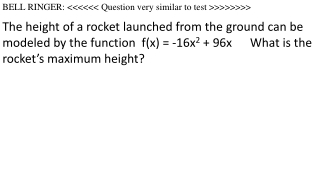DownloadDownload PresentationBELL RINGER: <<<<<< Question very similar to test >>>>>>>>

# BELL RINGER: <<<<<< Question very similar to test >>>>>>>>

Télécharger la présentation## BELL RINGER: <<<<<< Question very similar to test >>>>>>>>

- - - - - - - - - - - - - - - - - - - - - - - - - - - E N D - - - - - - - - - - - - - - - - - - - - - - - - - - -
##### Presentation Transcript

1. BELL RINGER: <<<<<< Question very similar to test >>>>>>>> The height of a rocket launched from the ground can be modeled by the function f(x) = -16x2 + 96x What is the rocket’s maximum height?

2. Test 1.2 Review Vertex form of a quadratic equation: f(x) = a(x – h)2 + k The vertex is the ordered pair (h,k) Standard form of a quadratic equation: f(x) = ax2 + bx + c Find the vertex when in standard form using x = -b/2a and y = f(-b/2a). The vertex provides the maximum or minimum value. Identify the axis of symmetry of a quadratic equation in standard form by finding the x value of the vertex. How to make a table when you have a quadratic equation in any form.

3. 2. The distance d in meters traveled by a skateboard on a ramp is related to the time traveled t in seconds. This is modeled by the function What is the maximum distance the skateboard can travel, and at what time would it achieve this distance? Round your answers to the nearest hundredth. a. 5.00 meters in 0 seconds c. 4.73 meters at 0.23 seconds b. 0.23 meters at 4.73 seconds d. 5.00 meters at 0.47 seconds

4. 4. The function gives the height in feet of a ball seconds after it is thrown upward from the roof of a 64-foot tall building. How many seconds after the ball is thrown does it reach its maximum height? What is the ball’s maximum height? • The ball reaches a maximum height of 64 feet 0 seconds after it is thrown. • The ball reaches a maximum height of 96 feet 1 second after it is thrown. • The ball reaches a maximum height of 100 feet 1.5 seconds after it is thrown. • d. The ball reaches a maximum height of 104 feet 1.5 • seconds after it is thrown.

5. 5. A ball is thrown from the top of a hill. The graph shows the relationship between the height , in meters, of the ball above the ground at the base of the hill and the time, in seconds, after the ball is thrown. The function models as a function of . What is the significance of the vertex of the parabola in terms of height and/or time?

6. 7. Write the function f(x) = 6x2 – 24x + 21 in vertex form, and identify its vertex.

7. 8. Find the axis of symmetry of the parabola.

8. 9. Kristi rides her bike to school and has an odometer that measures the distance traveled. She subtracts this distance from the distance to the school and records the distance that remains between her and the school. Find the intercepts. What do the intercepts represent?

9. 10. The table shows the height of a sassafras tree at each of two ages. What was the tree’s average rate of growth during this time period?

10. 12. The graph shows the height , in feet, of a football at time , in seconds, from the moment it was kicked at ground level. Estimate the average rate of change in height from 1.5 seconds to 1.75 seconds.

11. Graph • Find the axis of symmetry and the vertex.

12. 14 Consider What is its vertex and y-intercept?

13. 15. Find the x- and y-intercepts of

14. 18. This table shows the U.S. federal minimum hourly wage in different years. During which time interval did the wage increase at the greatest rate?

15. Extra Study Guide Problem: The parent function f(x) = x2 is vertically stretched by a factor of 4 and translated 6 units right and 8 units up. Write the new equation in vertex and standard form.